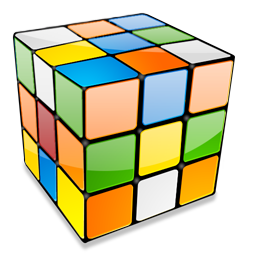#Combinatorics 2.0.0

.NET Standard 1.1 .NET Framework 4.6.1
`dotnet add package Combinatorics --version 2.0.0`
`NuGet\Install-Package Combinatorics -Version 2.0.0`
This command is intended to be used within the Package Manager Console in Visual Studio, as it uses the NuGet module's version of Install-Package.
`<PackageReference Include="Combinatorics" Version="2.0.0" />`
For projects that support PackageReference, copy this XML node into the project file to reference the package.
`paket add Combinatorics --version 2.0.0`
`#r "nuget: Combinatorics, 2.0.0"`
#r directive can be used in F# Interactive and Polyglot Notebooks. Copy this into the interactive tool or source code of the script to reference the package.
```// Install Combinatorics as a Cake Addin

// Install Combinatorics as a Cake Tool
#tool nuget:?package=Combinatorics&version=2.0.0```

A combinatorics library providing combinations, permutations & variations. This nuget package wraps up Adrian Akison's original implementation. See: http://www.codeproject.com/Articles/26050/Permutations-Combinations-and-Variations-using-C-G

Product Compatible and additional computed target framework versions.
.NET net5.0 was computed.  net5.0-windows was computed.  net6.0 was computed.  net6.0-android was computed.  net6.0-ios was computed.  net6.0-maccatalyst was computed.  net6.0-macos was computed.  net6.0-tvos was computed.  net6.0-windows was computed.  net7.0 was computed.  net7.0-android was computed.  net7.0-ios was computed.  net7.0-maccatalyst was computed.  net7.0-macos was computed.  net7.0-tvos was computed.  net7.0-windows was computed.
.NET Core netcoreapp1.0 was computed.  netcoreapp1.1 was computed.  netcoreapp2.0 was computed.  netcoreapp2.1 was computed.  netcoreapp2.2 was computed.  netcoreapp3.0 was computed.  netcoreapp3.1 was computed.
.NET Standard netstandard1.1 is compatible.  netstandard1.2 was computed.  netstandard1.3 was computed.  netstandard1.4 was computed.  netstandard1.5 was computed.  netstandard1.6 was computed.  netstandard2.0 is compatible.  netstandard2.1 was computed.
.NET Framework net45 was computed.  net451 was computed.  net452 was computed.  net46 was computed.  net461 is compatible.  net462 was computed.  net463 was computed.  net47 was computed.  net471 was computed.  net472 was computed.  net48 was computed.  net481 was computed.
MonoAndroid monoandroid was computed.
MonoMac monomac was computed.
MonoTouch monotouch was computed.
Tizen tizen30 was computed.  tizen40 was computed.  tizen60 was computed.
Universal Windows Platform uap was computed.  uap10.0 was computed.
Windows Phone wpa81 was computed.
Windows Store netcore was computed.  netcore45 was computed.  netcore451 was computed.
Xamarin.iOS xamarinios was computed.
Xamarin.Mac xamarinmac was computed.
Xamarin.TVOS xamarintvos was computed.
Xamarin.WatchOS xamarinwatchos was computed.
Compatible target framework(s)
• #### .NETFramework 4.6.1

• No dependencies.

• #### .NETStandard 2.0

• No dependencies.

### NuGet packages (4)

Showing the top 4 NuGet packages that depend on Combinatorics:

IIR_Butterworth_CS_Library

C# code to calculate the coefficients of the Butterworth filter and to filter the data This code calculates the coefficients of the Band-pass, Band-stop, Low-pass and High-pass Butterworth filters. It also filters the data, but no zero-phase delay is applied. The namespace is IIR_Butterworth_CS_Library. Each filter function will return a 2 rows x N coefficients 2D vector, where Row 1 = Numerator and Row 2 = Denumerator. The method "Check_stability_iir" can be used to check the stability of the filter. Please, keep in mind that if the filter is unstable, numerical instability leading to numerical overflow might happen when the order selected is extremely high. If that situation occurs, the program might assign a default value of 10^10 at the denominator. Band-pass: the function is "double[][] Lp2bp(double W_f1, double W_f2, int order_filt)". The first two arguments are the two normalized cut-off frequencies (f1/NF, f2/NF), where NF is the Nyquist frequency. This means that the cutoff frequencies must be within the interval of (0,1). The last argument is the order. Please, keep in mind that if you enter order_filt = 2, the order of the order will be 2 * order_filt = 4; Band-stop: the function is "double[][] Lp2bs(double W_f1, double W_f2, int order_filt)". The first two arguments are the two normalized cut-off frequencies (f1/NF, f2/NF), where NF is the Nyquist frequency. This means that the cutoff frequencies must be within the interval of (0,1). The last argument is the order. Please, keep in mind that if you enter order_filt = 2, the order of the order will be 2 * order_filt = 4; Low-pass: the function is "double[][] Lp2hp(double W_f1, int order_filt)". The first argument is the normalized cut-off frequency (f/NF), where NF is the Nyquist frequency. This means that the cutoff frequency must be within the interval of (0,1). The last argument is the order; High-pass: the function is "double[][] Lp2lp(double W_f1, int order_filt)". The first argument is the normalized cut-off frequency (f/NF), where NF is the Nyquist frequency. This means that the cutoff frequency must be within the interval of (0,1). The last argument is the order; Check the stability of the filter: the method is "bool Check_stability_iir(double[][] coeff_filt)". The argument is the 2D array containing the filter coefficients. It returns "true" if the filter is stable, "false" if it is unstable. Filter the data: the method is "double[] Filter_Data(double[][] coeff_filt, double[] pre_filt_signal)". The two arguments are the filter coefficients and the signal to be filtered. It returns the filtered signal. This code has been written following the Matlab code, so the arguments of each function reflect the arguments that you should pass to the equivalent functions in Matlab. I tried to be consistent with the names of the functions, in case someone wants to compare this code with Matlab code. The only exception is the function Check_stability_iir, which is not present in Matlab. If you have any question and/or want to report bugs, please e-mail me (Ale) at: pressalex@hotmail.com

Skunked

A library of classes to model the game of Cribbage.

MNCD

Multi-layer Network Community Detection

Net.Math

Simple but handful math functions

### GitHub repositories (1)

Showing the top 1 popular GitHub repositories that depend on Combinatorics:

Repository Stars
Illumina/canvas
Canvas - Copy number variant (CNV) calling from DNA sequencing data